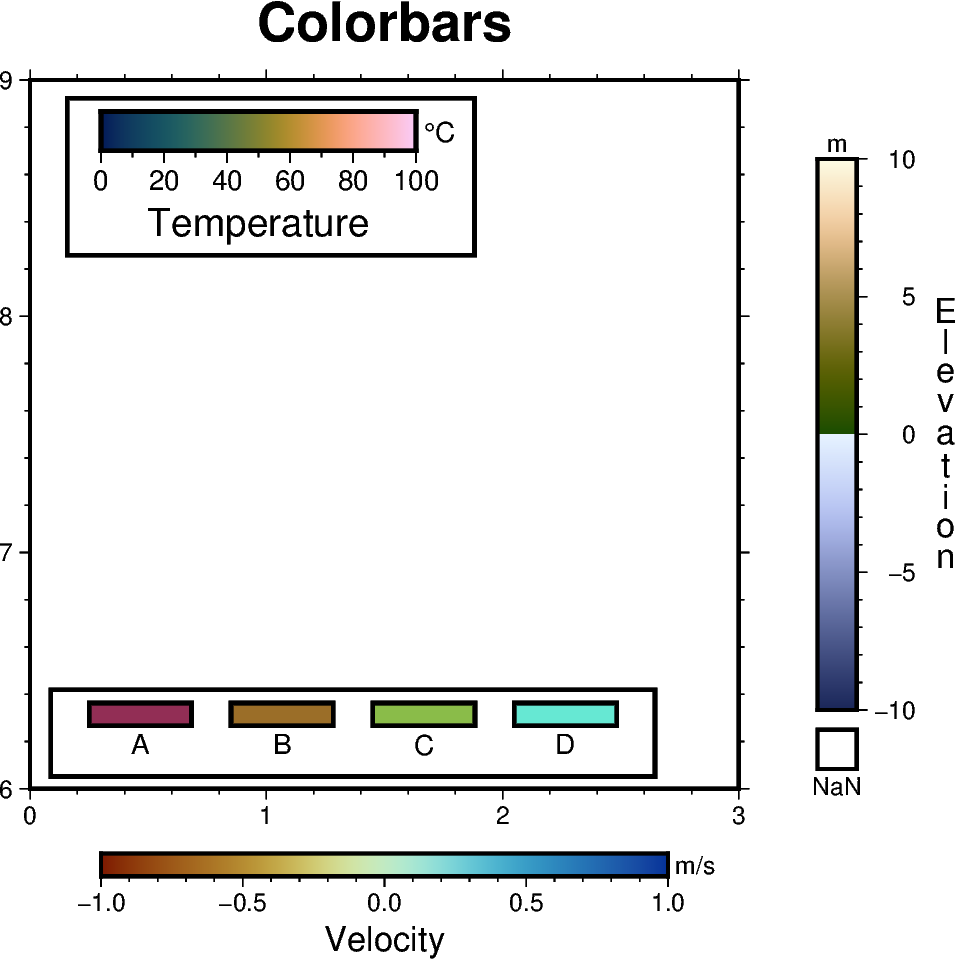# Colorbar

The `pygmt.Figure.colorbar` method creates a color scalebar. We must specify the colormap via the `cmap` parameter, and optionally set the placement via the `position` parameter. The full list of color palette tables can be found at https://docs.generic-mapping-tools.org/latest/cookbook/cpts.html. You can set the `position` of the colorbar using the following options:

• j/J: placed inside/outside the plot bounding box using any 2-character combination of vertical (Top, Middle, Bottom) and horizontal (Left, Center, Right) alignment codes, e.g. `position="jTR"` for Top Right.

• g: using map coordinates, e.g. `position="g170/-45"` for longitude 170E, latitude 45S.

• x: using paper coordinates, e.g. `position="x5c/7c"` for 5 cm, 7 cm from anchor point.

• n: using normalized (0-1) coordinates, e.g. `position="n0.4/0.8"`.

Note that the anchor point defaults to the bottom left (BL). Append `+h` to `position` to get a horizontal colorbar instead of a vertical one.```import pygmt

fig = pygmt.Figure()
fig.basemap(region=[0, 3, 6, 9], projection="x3c", frame=["af", "WSne+tColorbars"])

# ============
# Create a colorbar designed for seismic tomography - roma
# Colorbar is placed at bottom center (BC) by default if no position is given
fig.colorbar(cmap="roma", frame=["x+lVelocity", "y+lm/s"])

# ============
# Create a colorbar showing the scientific rainbow - batlow
fig.colorbar(
cmap="batlow",
# Colorbar positioned at map coordinates (g) longitude/latitude 0.3/8.7,
# with a length/width (+w) of 4 cm by 0.5 cm, and plotted horizontally (+h)
position="g0.3/8.7+w4c/0.5c+h",
box=True,
frame=["x+lTemperature", r"y+l\260C"],
scale=100,
)

# ============
# Create a colorbar suitable for surface topography - oleron
fig.colorbar(
cmap="oleron",
# Colorbar placed outside the plot bounding box (J) at Middle Right (MR),
# offset (+o) by 1 cm horizontally and 0 cm vertically from anchor point,
# with a length/width (+w) of 7 cm by 0.5 cm and a box for NaN values (+n)
position="JMR+o1c/0c+w7c/0.5c+n+mc",
# Note that the label 'Elevation' is moved to the opposite side and plotted
# vertically as a column of text using '+mc' in the position parameter
# above
frame=["x+lElevation", "y+lm"],
scale=10,
)

# ============
# Create a colorbar suitable for categorical data - hawaii
# Set up the colormap
pygmt.makecpt(
cmap="hawaii",
series=[0, 3, 1],
# Comma-separated string for the annotations of the colorbar
color_model="+cA,B,C,D",
)
# Plot the colorbar
fig.colorbar(
cmap=True,  # Use colormap set up above
# Colorbar placed inside the plot bounding box (j) at Bottom Left (BL),
# offset (+o) by 0.5 cm horizontally and 0.8 cm vertically from anchor
# point, and plotted horizontally (+h)
position="jBL+o0.5c/0.8c+h",
box=True,
# Divide colorbar into equal-sized rectangles
equalsize=0.5,
)

fig.show()
```

Total running time of the script: ( 0 minutes 1.039 seconds)

Gallery generated by Sphinx-Gallery